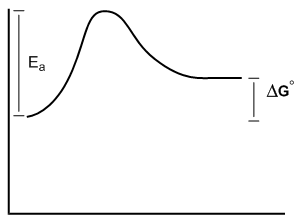Clutch Prep is now a part of Pearson
Ch. 6 - Thermodynamics and KineticsWorksheetSee all chapters

# Energy Diagram

See all sections
Sections
Energy Diagram
Gibbs Free Energy
Enthalpy
Entropy
Hammond Postulate
Carbocation Stability
Carbocation Intermediate Rearrangements

You might have heard of the terms thermodynamics and kinetics before, but in chemistry they mean something very specific. Free energy diagrams are going to give us a snapshot of what these terms mean.

Concept #1: Introduction to free energy diagrams.

Transcript

When talking about thermal dynamics and kinetics, one of our best friends is going to be the free-energy diagram. So it's going to be essential that we learn how to interpret these correctly. The reason free-energy diagrams are important is because they're going to serve as a summary of the thermal dynamics and kinetics of a reaction. So what I want to do first is relate free-energy diagrams to what we've already learned and then learn how to interpret them.
So remember that in chapter one, maybe you guys were able to watch that lesson, maybe you didn't, but it's fine. Remember that atoms save energy by forming bonds. The entire idea of a chemical bond is it's a shared region of space that they share electrons. And remember that we could use molecular orbital diagrams to really illustrate how much energy we were saving.
So remember that we had our atomic orbitals on the sides and we had our molecular orbitals on the top and the bottom. And even if you don't remember this that well, that's okay because I'm just going to show you that, for example, for a hydrogen atom remember that a hydrogen atom over here would have one electron. A hydrogen atom over here would have another electron. These are called 1s because remember that they just fill the 1s orbital.
This is what they would look like in terms of energy if they were not bonded to anything. Remember that by sharing electrons, though, they could wind up filling their octet and filling their atomic orbital. So what we said was that as you make a bond between these two hydrogens, you're going to jump down in energy. And that's what this would look like. It would look like this after they're bonded because now you're saving energy by sharing those electrons.
Now remember that we could also use a graph of the distances to figure out how much energy we're saving as the nuclei got closer together. So here, just so you guys know, this was the energy state that we had over here if it was not bonded to anything. And you can see these hydrogen atoms the way that I drew them here, they're not bonded. But then, as we get them closer together, as we get our nuclei closer together, what would eventually happen is that they would form this perfect sweet spot where they'd be the perfect distance apart, in this case, it was 1.33 angstroms, and that would be the amount of distance required to save the maximum amount of energy.
Now the number of the energy isn't important. This just happened to be negative 436 kilojoules per mole. You don't need to memorize that at all. Later on, we're going to use a chart to figure that out. But what is important is that all this information can be related on the free-energy diagram. So the free-energy diagram is basically a storyline of everything that I just said.
What it tells us is that this is what the atoms looked like before they were bonded. This was their energy level. After they decided to react, this is what they looked like and now this is our energy level. So did we save energy or did we spend energy? That's really the way we think about it. And in this case, we saved energy. And it's going to be the same amount that we were talking about over here.
That's what we do with a free-energy diagram. What we're looking at is the x-axis is the reaction coordinates, saying as the reaction proceeds, what are the entities looking like. And then the y-axis is usually going to be either heat, talking about enthalpy or it's going to be spontaneity, which we're going to talk about in just a second, which is the delta G.
So here I'm just going to go ahead and restate some stuff. Free-energy diagrams give us information on spontaneity and rate of reactions. And now what does this mean? What it means is that this has to do with thermal dynamics and kinetics. Thermal dynamics is what we call spontaneity and that describes the favorability of a reaction. So when I say that something's spontaneous that means that it's favorable. That means that it wants to happen by itself.
The equation that we use to understand thermodynamics is going to be, your favorite, Gibbs free energy. We can't get away from this equation. We're going to be using it pretty much for most of this chapter Remember what it was was that delta G was equal to delta H, which is the enthalpy, minus the temperature times the entropy or the delta S. Later on, I'm going to be going in-depth on each of these variables. But for right now just know that that's what delta G is. It's spontaneity.
Delta G is usually related by the difference in energy between the beginning and the end. That's usually the delta G. Then what's the rate or the kinetics? The kinetics has to do with how fast the reaction would take place if it is favorable or even it's not favorable how fast would this reaction take place. There are a lot of very spontaneous reactions in this world that do not happen at measurable rates or in appreciable rates because they're so slow.
The kinetics has to do with the activation energy that it takes in order to make a reaction go forward. The activation energy in the free-energy diagram I gave you above would be the difference in the energy between the beginning and your highest point in the reaction. This would be my activation energy. Later on we're going to describe that better as well, but for right now just know that it's basically the difference between where you started and the highest point you have to achieve in order to make the reaction go forward.
So what I want to do now is I want to just do some really basic qualitative recognition here. This isn't about – I don't want you guys to calculate anything yet. We're just going to decide what kind of reactions are we looking at here. Are they going to be spontaneous? Are they going to be non-spontaneous? Are they going to happen fast? Are they going to happen slow?
So what I want you guys to do is go ahead and look at the following four reactions and try to figure out if it's spontaneous or not and if the rate is going to happen quickly or slowly or fast or slow and then I'll go ahead and answer these for you. All right, so let's go.

Atoms save energy by forming bonds. Free energy diagrams show overall changes in potential energy during reactions.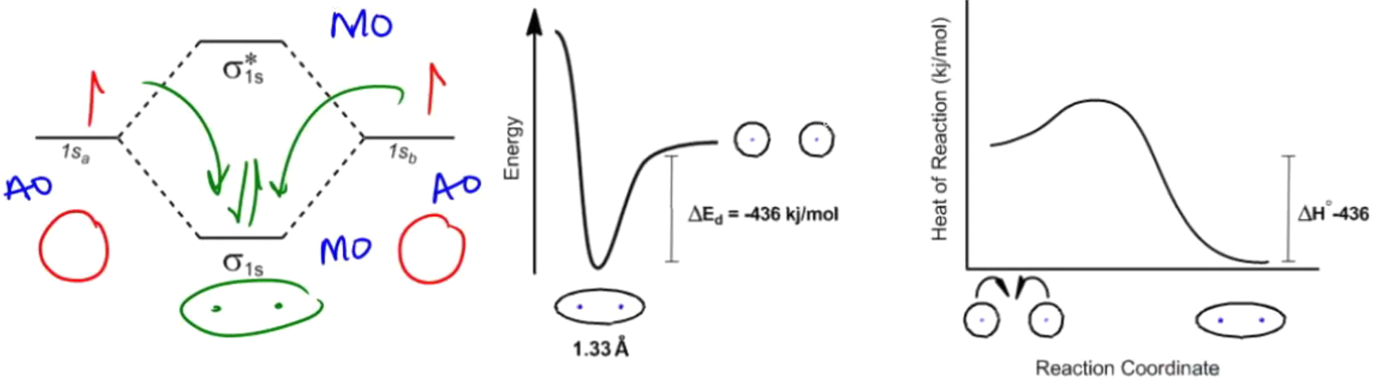Free energy diagrams give us information on the spontaneity and rate of reactions:

• Thermodynamics describes favorability. Determined by Gibbs Free Energy (ΔG° = ΔH° - TΔS).
• Kinetics describes rate. Determined by the activation energy of the rate determining step (Ea).

Example #1: Describe the favorability and rate of the following free energy diagram.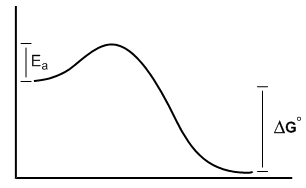Example #2: Describe the favorability and rate of the following free energy diagram.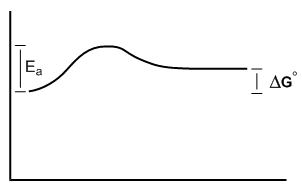Example #3: Describe the favorability and rate of the following free energy diagram.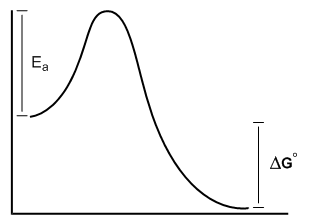Example #4: Describe the favorability and rate of the following free energy diagram.